# locallapfilt

Fast local Laplacian filtering of images

## Syntax

``B = locallapfilt(I,sigma,alpha)``
``B = locallapfilt(I,sigma,alpha,beta)``
``B = locallapfilt(___,Name,Value)``

## Description

example

````B = locallapfilt(I,sigma,alpha)` filters the grayscale or RGB image `I` with an edge-aware, fast local Laplacian filter. `sigma` characterizes the amplitude of edges in `I`. `alpha` controls smoothing of details.```
````B = locallapfilt(I,sigma,alpha,beta)` filters the image using `beta` to control the dynamic range of `A`.```

example

````B = locallapfilt(___,Name,Value)` uses name-value pairs to control advanced aspects of the filter.```

## Examples

collapse all

Import an RGB image

`A = imread('peppers.png');`

Set parameters of the filter to increase details smaller than 0.4.

```sigma = 0.4; alpha = 0.5;```

Use fast local Laplacian filtering

`B = locallapfilt(A, sigma, alpha);`

Display the original and filtered images side-by-side.

`imshowpair(A, B, 'montage')`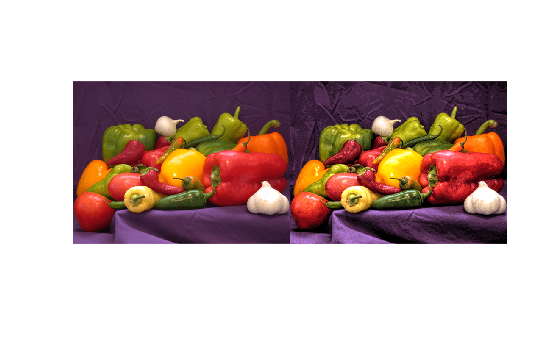Local Laplacian filtering is a computationally intensive algorithm. To speed up processing, `locallapfilt` approximates the algorithm by discretizing the intensity range into a number of samples defined by the '`NumIntensityLevels`' parameter. This parameter can be used to balance speed and quality.

Import an RGB image and display it.

```A = imread('peppers.png'); figure imshow(A) title('Original Image')```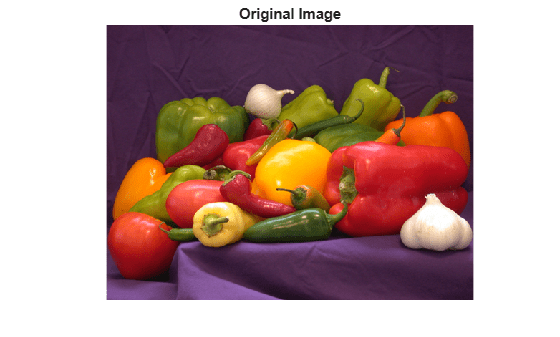Use a `sigma` value to process the details and an `alpha` value to increase the contrast, effectively enhancing the local contrast of the image.

```sigma = 0.2; alpha = 0.3;```

Using fewer samples increases the execution speed, but can produce visible artifacts, especially in areas of flat contrast. Time the function using only 20 intensity levels.

`t_speed = timeit(@() locallapfilt(A, sigma, alpha, 'NumIntensityLevels', 20)) `
```t_speed = 0.1629 ```

Now, process the image and display it.

```B_speed = locallapfilt(A, sigma, alpha, 'NumIntensityLevels', 20); figure imshow(B_speed) title(['Enhanced with 20 intensity levels in ' num2str(t_speed) ' sec'])```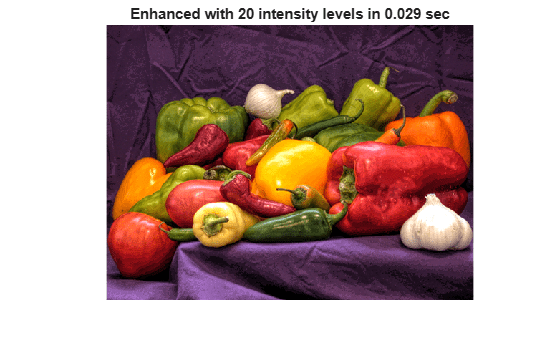A larger number of samples yields better looking results at the expense of more processing time. Time the function using 100 intensity levels.

`t_quality = timeit(@() locallapfilt(A, sigma, alpha, 'NumIntensityLevels', 100))`
```t_quality = 0.7390 ```

Process the image with 100 intensity levels and display it:

```B_quality = locallapfilt(A, sigma, alpha, 'NumIntensityLevels', 100); figure imshow(B_quality) title(['Enhancement with 100 intensity levels in ' num2str(t_quality) ' sec'])```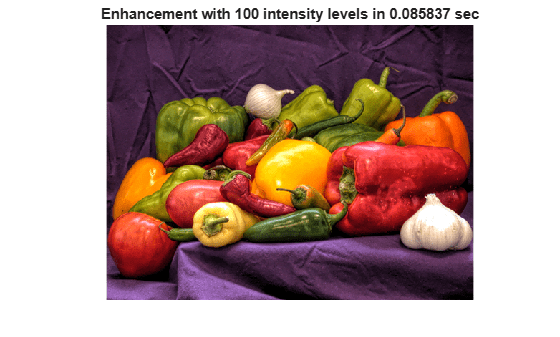Try varying the number of intensity levels on your own images. Try also flattening the contrast (with `alpha` > 1). You will see that the optimal number of intensity levels is different for every image and varies with `alpha`. By default, `locallapfilt` uses a heuristic to balance speed and quality, but it cannot predict the best value for every image.

Import a color image, reduce its size, and display it.

```A = imread('car2.jpg'); A = imresize(A, 0.25); figure imshow(A) title('Original Image')```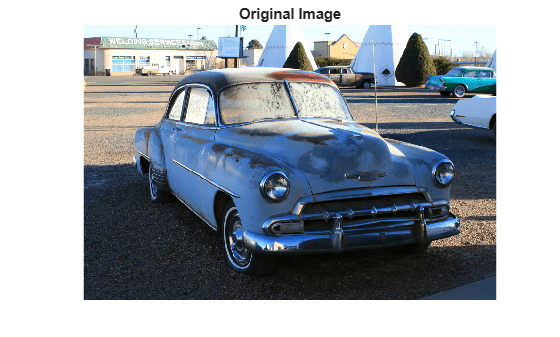Set the parameters of the filter to dramatically increase details smaller than 0.3 (out of a normalized range of 0 to 1).

```sigma = 0.3; alpha = 0.1;```

Let's compare the two different modes of color filtering. Process the image by filtering its intensity and by filtering each color channel separately:

```B_luminance = locallapfilt(A, sigma, alpha); B_separate = locallapfilt(A, sigma, alpha, 'ColorMode', 'separate');```

Display the filtered images.

```figure imshow(B_luminance) title('Enhanced by boosting the local luminance contrast')```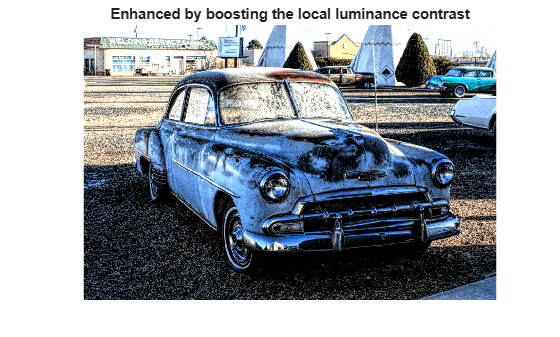```figure imshow(B_separate) title('Enhanced by boosting the local color contrast')```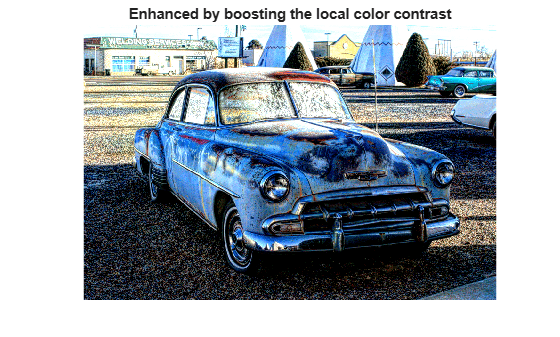An equal amount of contrast enhancement has been applied to each image, but colors are more saturated when setting '`ColorMode`' to '`separate`'.

Import an image. Convert the image to floating point so that we can add artificial noise more easily.

```A = imread('pout.tif'); A = im2single(A);```

Add Gaussian noise with zero mean and 0.001 variance.

```A_noisy = imnoise(A, 'gaussian', 0, 0.001); psnr_noisy = psnr(A_noisy, A); fprintf('The peak signal-to-noise ratio of the noisy image is %0.4f\n', psnr_noisy); ```
```The peak signal-to-noise ratio of the noisy image is 30.0234 ```

Set the amplitude of the details to smooth, then set the amount of smoothing to apply.

```sigma = 0.1; alpha = 4.0;```

Apply the edge-aware filter.

```B = locallapfilt(A_noisy, sigma, alpha); psnr_denoised = psnr(B, A); fprintf('The peak signal-to-noise ratio of the denoised image is %0.4f\n', psnr_denoised);```
```The peak signal-to-noise ratio of the denoised image is 32.2016 ```

Note an improvement in the PSNR of the image.

Display all three images side by side. Observe that details are smoothed and sharp intensity variations along edges are unchanged.

```figure subplot(1,3,1), imshow(A), title('Original') subplot(1,3,2), imshow(A_noisy), title('Noisy') subplot(1,3,3), imshow(B), title('Denoised')```Import the image, resize it and display it

```A = imread('car1.jpg'); A = imresize(A, 0.25); figure imshow(A) title('Original Image')```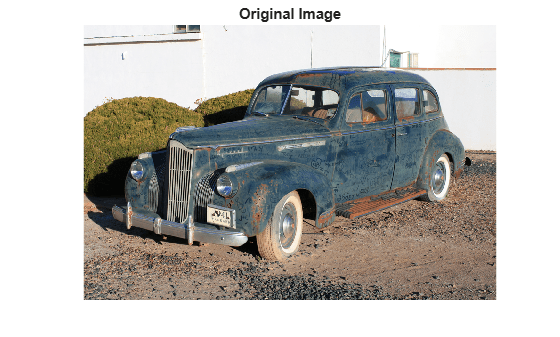The car is dirty and covered in markings. Let's try to erase the dust and markings on the body. Set the amplitude of the details to smooth, and set a large amount of smoothing to apply.

```sigma = 0.2; alpha = 5.0;```

When smoothing (`alpha` > 1), the filter produces high quality results with a small number of intensity levels. Set a small number of intensity levels to process the image faster.

`numLevels = 16;`

Apply the filter.

`B = locallapfilt(A, sigma, alpha, 'NumIntensityLevels', numLevels);`

Display the "clean" car.

```figure imshow(B) title('After smoothing details')```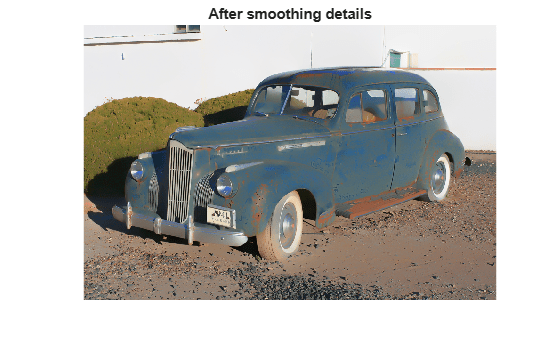## Input Arguments

collapse all

Image to filter, specified as a 2-D grayscale image or 2-D truecolor image.

Data Types: `single` | `int8` | `int16` | `uint8` | `uint16`

Amplitude of edges, specified as a non-negative number. `sigma` should be in the range [0, 1] for integer images and for single images defined over the range [0, 1]. For single images defined over a different range [`a`, `b`], `sigma` should also be in the range [`a`, `b`].

Data Types: `single` | `double` | `int8` | `int16` | `int32` | `int64` | `uint8` | `uint16` | `uint32` | `uint64`

Smoothing of details, specified as a positive number. Typical values of `alpha` are in the range [0.01, 10].

ValueDescription
`alpha` less than `1`Increases the details of the input image, effectively enhancing the local contrast of the image without affecting edges or introducing halos.
`alpha` greater than `1`Smooths details in the input image while preserving crisp edges
`alpha` equal to `1`The details of the input image are left unchanged.

Data Types: `single` | `double` | `int8` | `int16` | `int32` | `int64` | `uint8` | `uint16` | `uint32` | `uint64`

Dynamic range, specified as a non-negative number. Typical values of beta are in the range [0, 5]. `beta` affects the dynamic range of `A`.

ValueDescription
`beta` less than `1`Reduces the amplitude of edges in the image, effectively compressing the dynamic range without affecting details.
`beta` greater than `1`Expands the dynamic range of the image.
`beta` equal to `1`Dynamic range of the image is left unchanged. This is the default value.

Data Types: `single` | `double` | `int8` | `int16` | `int32` | `int64` | `uint8` | `uint16` | `uint32` | `uint64`

### Name-Value Arguments

Specify optional comma-separated pairs of `Name,Value` arguments. `Name` is the argument name and `Value` is the corresponding value. `Name` must appear inside quotes. You can specify several name and value pair arguments in any order as `Name1,Value1,...,NameN,ValueN`.

Example: `'ColorMode','separate'`

Method used to filter RGB images, specified as one of the following values. This parameter has no effect on grayscale images.

ValueDescription
`'luminance'``locallapfilt` converts the input RGB image to grayscale before filtering and reintroduces color after filtering, which changes the contrast of the input image without affecting colors.
`'separate'``locallapfilt` filters each color channel independently.

Data Types: `char` | `string`

Number of intensity samples in the dynamic range of the input image, specified as `'auto'` or positive integer. A higher number of samples gives results closer to exact local Laplacian filtering. A lower number increases the execution speed. Typical values are in the range `[10, 100]`. If set to `'auto'`, `locallapfilt` chooses the number of intensity levels automatically to balance quality and speed based on other parameters of the filter.

Data Types: `single` | `double` | `int8` | `int16` | `int32` | `int64` | `uint8` | `uint16` | `uint32` | `uint64` | `char` | `string`

## Output Arguments

collapse all

Filtered image, returned as a numeric array the same size and data type as the input image, `A`.

 Paris, Sylvain, Samuel W. Hasinoff, and Jan Kautz. Local Laplacian filters: edge-aware image processing with a Laplacian pyramid, ACM Trans. Graph. 30.4 (2011): 68.

 Aubry, Mathieu, et al. Fast local laplacian filters: Theory and applications. ACM Transactions on Graphics (TOG) 33.5 (2014): 167.